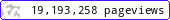•••••MadAsMaths.com :: I.Y.G.B. Practice Papers :: A Level Practice Papers 2019 Spec :: SYN Practice Papers

I.Y.G.B. SYN PRACTICE PAPERS

These I.Y.G.B. synoptic practice papers are suitable for the new A Level Pure Mathematics specification, introduced for teaching from 2017.
They do not follow any particular board syllabus; instead they are a synopsis for the first two Pure Mathematics modules.

They will offer good practice for able mathematics students as they will be generally more difficult than the standard "A to R" difficulty of the MP1/MP2 range.

They will also be suitable for students taking A level Mathematics from all boards.

All papers have model solutions.

 syn_a.pdfsyn_a_solutions.pdfsyn_b.pdfsyn_b_solutions.pdfsyn_c.pdfsyn_c_solutions.pdfsyn_d.pdfsyn_d_solutions.pdfsyn_e.pdfsyn_e_solutions.pdfsyn_f.pdfsyn_f_solutions.pdfsyn_g.pdfsyn_g_solutions.pdfsyn_h.pdfsyn_h_solutions.pdfsyn_i.pdfsyn_i_solutions.pdfsyn_j.pdfsyn_j_solutions.pdfsyn_k.pdfsyn_k_solutions.pdfsyn_l.pdfsyn_l_solutions.pdfsyn_m.pdfsyn_m_solutions.pdfsyn_n.pdfsyn_n_solutions.pdfsyn_o.pdfsyn_o_solutions.pdfsyn_p.pdfsyn_p_solutions.pdfsyn_q.pdfsyn_q_solutions.pdfsyn_r.pdfsyn_r_solutions.pdfsyn_s.pdfsyn_s_solutions.pdfsyn_t.pdfsyn_t_solutions.pdfsyn_u.pdfsyn_u_solutions.pdfsyn_v.pdfsyn_v_solutions.pdfsyn_w.pdfsyn_w_solutions.pdfsyn_x.pdfsyn_x_solutions.pdfsyn_y.pdfsyn_y_solutions.pdfsyn_z.pdfsyn_z_solutions.pdf